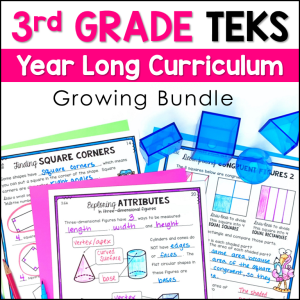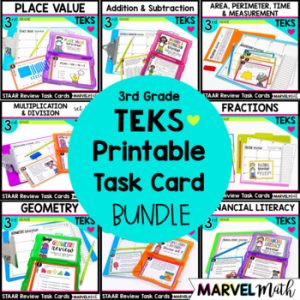Save 10% on your first order with code EXTRA10

# 3rd Grade Fractions TEKS STAAR Review Task Cards – Print

\$4.00\$296.75\$18.00
SKU: 7214039 Categories: ,

## Description

This resource includes 24 TEKS-aligned Printable Task Cards for Fractions. Topics covered include fractions on a number line, equivalent fractions, comparing fractions, and reasoning about fractions.

Find the digital Google Form version HERE. Both versions have the same questions.

The questions are rigorous and have bright graphics to keep students engaged. All questions are multiple choice and are STAAR formatted. I have designed these task cards to give more weight to the Readiness Standards which comprise nearly 2/3 of the STAAR test. The questions are rigorous and have bright graphics to keep students engaged. All questions are multiple choice.

You will love the diagnostic key that helps you easily identify student needs and form reteach groups quickly while you grade student work. The questions are rigorous and include convincing distractors (wrong answer choices), making these task cards a great tool in not only reviewing content but also giving you the opportunity to teach test-taking strategies.

What’s Included:

• 24 Task Cards
• Color and Blackline versions
• Color-coded diagnostic key to help you make re-teach groups based on the TEKS your students haven’t mastered.
• Task card sized mini-cover and a mini-key to help with organization
• Student Recording Sheets

Great ways to use these Task Cards:

• Math Station
• Guide a Small Group Lesson
• Whole Class Scoot – Students take their recording sheet to task cards posted around the room to solve each problem
• Tutoring Sessions
• End of Unit Review
• Collect Quantitative Data for Parent-Teacher Conferences or PLC and RTI Meetings
• STAAR Review

Topics include:

• Representing Fractions with Models
• Fractions on a Number Line
• Explaining Unit Fractions
• Representing Equivalent Fractions with Models and on Number Lines
• Comparing Fractions
• Composing and Decomposing Fractions into unit fractions (3/4 = 1/4 + 1/4 + 1/4)
• Dividing Objects into Fractional Shares

TEKS Alignment:

3.3F: (5 questions) Represent equivalent fractions with denominators of 2, 3, 4, 6, and 8 using a variety of objects and pictorial models, including number lines.

3.3H: (5 questions) Compare two fractions having the same numerator or denominator in problems by reasoning about their sizes and justifying the conclusion using symbols, words, objects, and pictorial models.

Supporting Standards

3.3A: (2 questions) Represent fractions greater than zero and less than or equal to one with denominators of 2, 3, 4, 6, and 8 using concrete objects and pictorial models, including strip diagrams and number lines.

3.3B: (2 questions) Determine the corresponding fraction greater than zero and less than or equal to one with denominators of 2, 3, 4, 6, and 8 given a specified point on a number line.

3.3C: (2 questions) Explain that the unit fraction 1/b represents the quantity formed by one part of a whole that has been partitioned into b equal parts where b is a non-zero whole number.

3.3D: (2 questions) Compose and decompose a fraction a/b with a numerator greater than zero and less than or equal to b as a sum of parts 1/b.

3.3E: (2 questions) Solve problems involving partitioning an object or a set of objects among two or more recipients using pictorial representations of fractions with denominators of 2, 3, 4, 6, and 8.

3.3G: (2 questions) Explain that two fractions are equivalent if and only if they are both represented by the same point on the number line or represent the same portion of a same size whole for an area model.